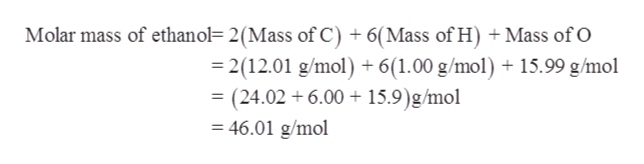# How many grams of ethanol (CH3CH2OH) are there in a 0.2814 mol sample?

Question
11 views

How many grams of ethanol (CH3CH2OH) are there in a 0.2814 mol sample?

check_circle

Step 1

It is given that there are 0.2814 moles of ethanol (CH3CH2OH).

Step 2

Molar mass of a substance is sum of atomic masses of all the individual atoms present in it. Mole is the amount of the substance that contains the same number of particles or atoms or molecules.

Number of moles can be expressed in terms of given mass and molar mass as follows:

Step 3

Molar mass of ethanol (CH3CH2OH) should be ...help_outlineImage TranscriptioncloseMolar mass of ethanol= 2(Mass of C) + 6(Mass of H) +Mass of O 2(12.01 g/mol) + 6(1.00 g/mol) 15.99 g/mol = (24.02 6.00 15.9)g/mol 46.01 g/mol fullscreen

### Want to see the full answer?

See Solution

#### Want to see this answer and more?

Solutions are written by subject experts who are available 24/7. Questions are typically answered within 1 hour.*

See Solution
*Response times may vary by subject and question.
Tagged in

### General Chemistry